Intro to Subtraction Math Video for Kids - Grades K-2
1%
It was processed successfully!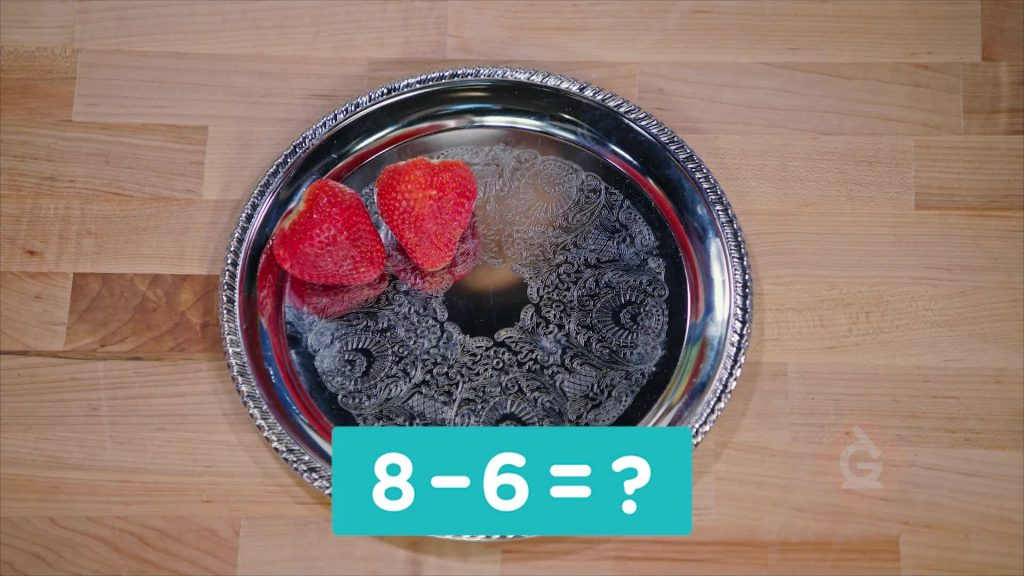WHAT IS SUBTRACTION?

Subtraction is taking away one group from a bigger group. When you subtract you are finding how many are left. You can subtract by counting how many are left. You can also subtract by counting back.

To better understand subtraction…

WHAT IS SUBTRACTION?. Subtraction is taking away one group from a bigger group. When you subtract you are finding how many are left. You can subtract by counting how many are left. You can also subtract by counting back. To better understand subtraction…

## LET’S BREAK IT DOWN!

### Count to find how many cars in all.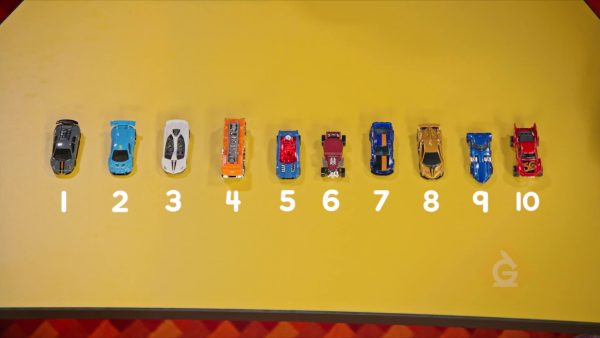Counting tells you how many you have in all. There are toy cars on a table. Say one number for each car. 1, 2, 3, 4, 5, 6, 7, 8, 9, 10. There are 10 cars on the table.

Count to find how many cars in all. Counting tells you how many you have in all. There are toy cars on a table. Say one number for each car. 1, 2, 3, 4, 5, 6, 7, 8, 9, 10. There are 10 cars on the table.

### Count how many baby chicks are left.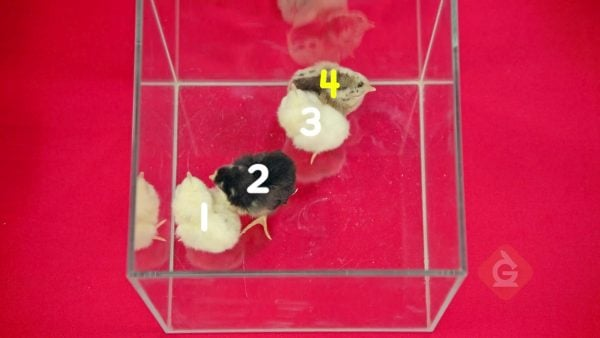There are baby chicks in a nest. Count the chicks to see how many in all. 1, 2, 3, 4, 5. There are 5 chicks in all. Sasha takes 1 chick out of the nest. How many chicks are left? 1, 2, 3, 4. There are 4 chicks left.

Count how many baby chicks are left. There are baby chicks in a nest. Count the chicks to see how many in all. 1, 2, 3, 4, 5. There are 5 chicks in all. Sasha takes 1 chick out of the nest. How many chicks are left? 1, 2, 3, 4. There are 4 chicks left.

### Use “–” and “=” to show subtraction.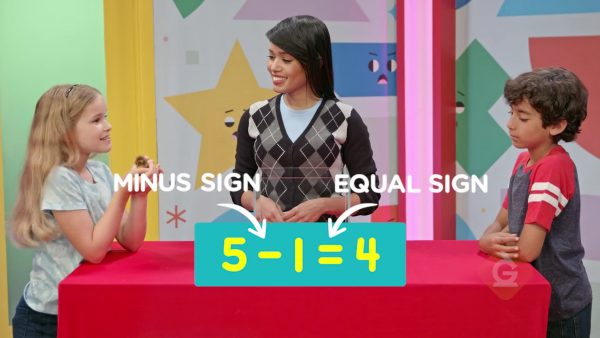A “–” shows a group being taken away from another group. 5 – 1 = 4. The “–” shows that 1 chick was taken away from a group of 5. The “=” shows that 5 takeaway 1 is equal to 4. There are 4 chicks left.

Use “–” and “=” to show subtraction. A “–” shows a group being taken away from another group. 5 – 1 = 4. The “–” shows that 1 chick was taken away from a group of 5. The “=” shows that 5 takeaway 1 is equal to 4. There are 4 chicks left.

### Use fingers to subtract donuts.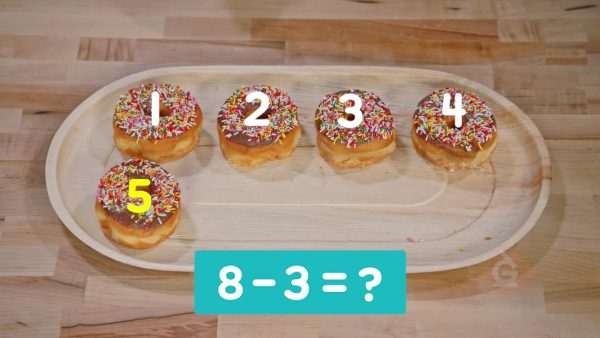You made 8 donuts. Your family at 3. How many donuts are left? Show 8 with your fingers. Put 3 fingers down. Count how many fingers you still have up:1, 2, 3, 4, 5. There are 5 donuts left. 8 – 3 = 5.

Use fingers to subtract donuts. You made 8 donuts. Your family at 3. How many donuts are left? Show 8 with your fingers. Put 3 fingers down. Count how many fingers you still have up:1, 2, 3, 4, 5. There are 5 donuts left. 8 – 3 = 5.

### Count back to subtract balloons.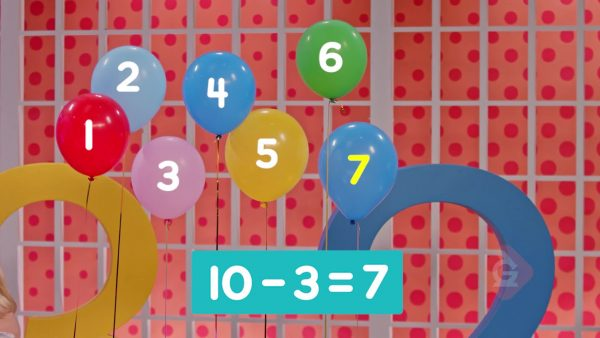There were 10 balloons. Then 3 balloons popped. How many balloons are left? Start at 10. Count back 3: 9, 8, 7. There are 7 balloons left. 10 – 3 = 7.

Count back to subtract balloons. There were 10 balloons. Then 3 balloons popped. How many balloons are left? Start at 10. Count back 3: 9, 8, 7. There are 7 balloons left. 10 – 3 = 7.

## INTRO TO SUBTRACTION VOCABULARY

To put together. If you add 3 and 2, you find how many there are in all.
Subtraction
To take away. If you take away 2 from 3, you find how many are left after 2 are taken away from 3.
Count on
To count in increasing counting order. 1, 2, 3, 4, 5, 6, …
Count back
To count in decreasing counting order. 9, 8, 7, 6, 5, …
Greater
A number is greater than another number if you count it second when counting.
Lesser
A number is less than another number if you count it first when counting.

## INTRO TO SUBTRACTION DISCUSSION QUESTIONS

### What does subtraction mean?

Subtraction means to take away. When I subtract 3 from 5, I take away 3 from 5.

### How can you use counters to show subtraction?

I can use counters to show how many there are to start. Then I can take away counters to show how many I subtract. Then I can count the amount that is left. Or I can count back from the start number as I take away the number of counters to subtract.

### Look at the number sentence 6 – 4 = 2. What does the – mean? What does the 2 mean?

The – means that 4 is taken away from 6. The 2 means that there are 2 left over. 6 take away 4 is 2.

### There are 4 balls in a bin. 1 is taken out. How many balls are left in the bin?

4 – 1 = 3. There are 3 balls left.

### How do you know what number to take away?

I always take away the smaller (lesser) number. The words in the problem tell me what number is there to start and what is taken away.
X

## Success

We’ve sent you an email with instructions how to reset your password.
Ok